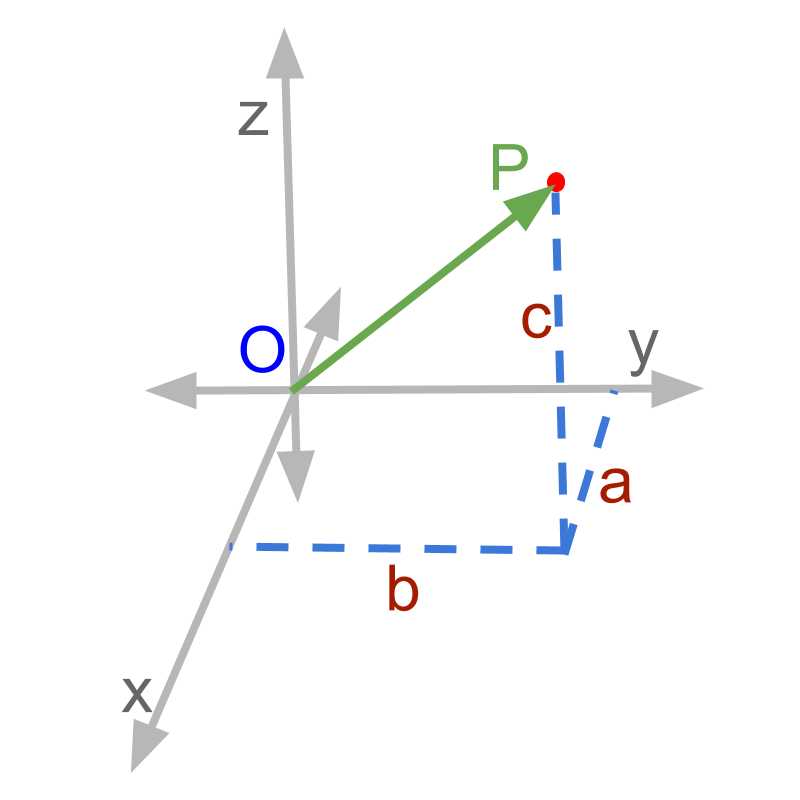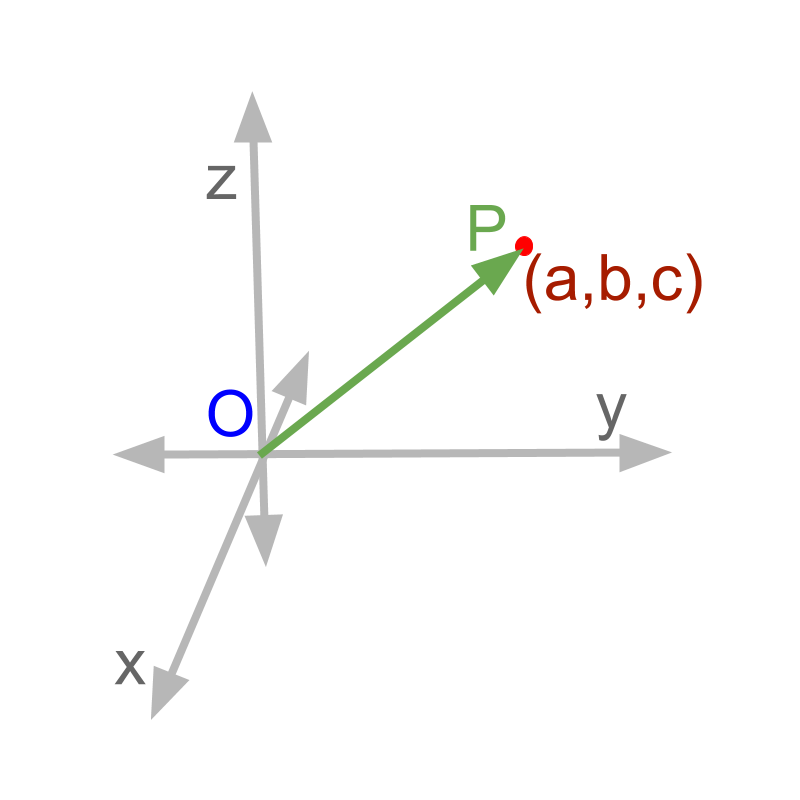maths > vector-algebra

Position Vector of a Point

what you'll learn...

Overview

Position Vector of a Point

»  For a point $P\left(a,b,c\right)$$P \left(a , b , c\right)$ the position vector is $\stackrel{\to }{OP}=ai+bj+ck$$\vec{O P} = a i + b j + c k$position means placeConsider a point $P$$P$ in 3D coordinate system as shown in figure. The point $P$$P$ is given by the following.

$\star$$\star$  in 3D coordinates it is $\left(a,b,c\right)$$\left(a , b , c\right)$

$\star$$\star$  as a vector representation $ai+bj+ck$$a i + b j + c k$

$\star$$\star$  as the vector $\stackrel{\to }{OP}$$\vec{O P}$

The word 'position' means 'place; location'.Given a point $P\left(a,b,c\right)$$P \left(a , b , c\right)$ as shown in figure. Position vector of a point $P\left(a,b,c\right)$$P \left(a , b , c\right)$ is
$\stackrel{\to }{OP}=ai+bj+ck$$\vec{O P} = a i + b j + c k$

Position Vector: For a point $P\left(a,b,c\right)$$P \left(a , b , c\right)$ the position vector is
$\stackrel{\to }{OP}=ai+bj+ck$$\vec{O P} = a i + b j + c k$.

Position Vector of a Point is the vector between origin and the point.

examples

What is the position vector of point $\left(2,-\frac{3}{4}\right)$$\left(2 , - \frac{3}{4}\right)$?

The answer is '$2i-\frac{3}{4}j$$2 i - \frac{3}{4} j$'.

Given the vector $\stackrel{\to }{OP}=3i-1.4j+2.5k$$\vec{O P} = 3 i - 1.4 j + 2.5 k$, if $O$$O$ is the origin, what is the coordinate position of point $P$$P$?

The answer is '$\left(3,-1.4,2.5\right)$$\left(3 , - 1.4 , 2.5\right)$'

What is the position vector of point $\left(0,-2.1,3.4\right)$$\left(0 , - 2.1 , 3.4\right)$?

The answer is '$-2.1j+3.4k$$- 2.1 j + 3.4 k$'.

summaryPosition Vector: For a point $P\left(a,b,c\right)$$P \left(a , b , c\right)$ the position vector is
$\stackrel{\to }{OP}=ai+bj+ck$$\vec{O P} = a i + b j + c k$.

Outline